www.dlr.de · Antonin RAFFIN · From Tabular Q-Learning to DQN · RL Summer School Barcelona · 27.06.2023

### From Tabular Q-Learning to DQN

Antonin RAFFIN ( @araffin2 )
German Aerospace Center (DLR)
https://araffin.github.io/

### GitHub Repository

#### Outline

1. RL 101 refresher
2. Tabular Q-Learning
3. RL as a regression problem (Fitted Q Iteration)
4. From FQI to Deep Q-Network (DQN)

### Stable-Baselines3 (SB3)


from stable_baselines3 import DQN
# SAC, TD3, TQC are all successors of DQN
from stable_baselines3 import SAC, TD3
from sb3_contrib import TQC

# Instantiate the algorithm on the Lunar Lander env
model = DQN("MlpPolicy", "LunarLander-v2", verbose=1)
# Train for 100 000 steps
model.learn(100_000, progress_bar=True)


### RL from scratch

Raffin et al. "Learning to Exploit Elastic Actuators for Quadruped Locomotion.": https://github.com/araffin/sbx

### Flappy Bird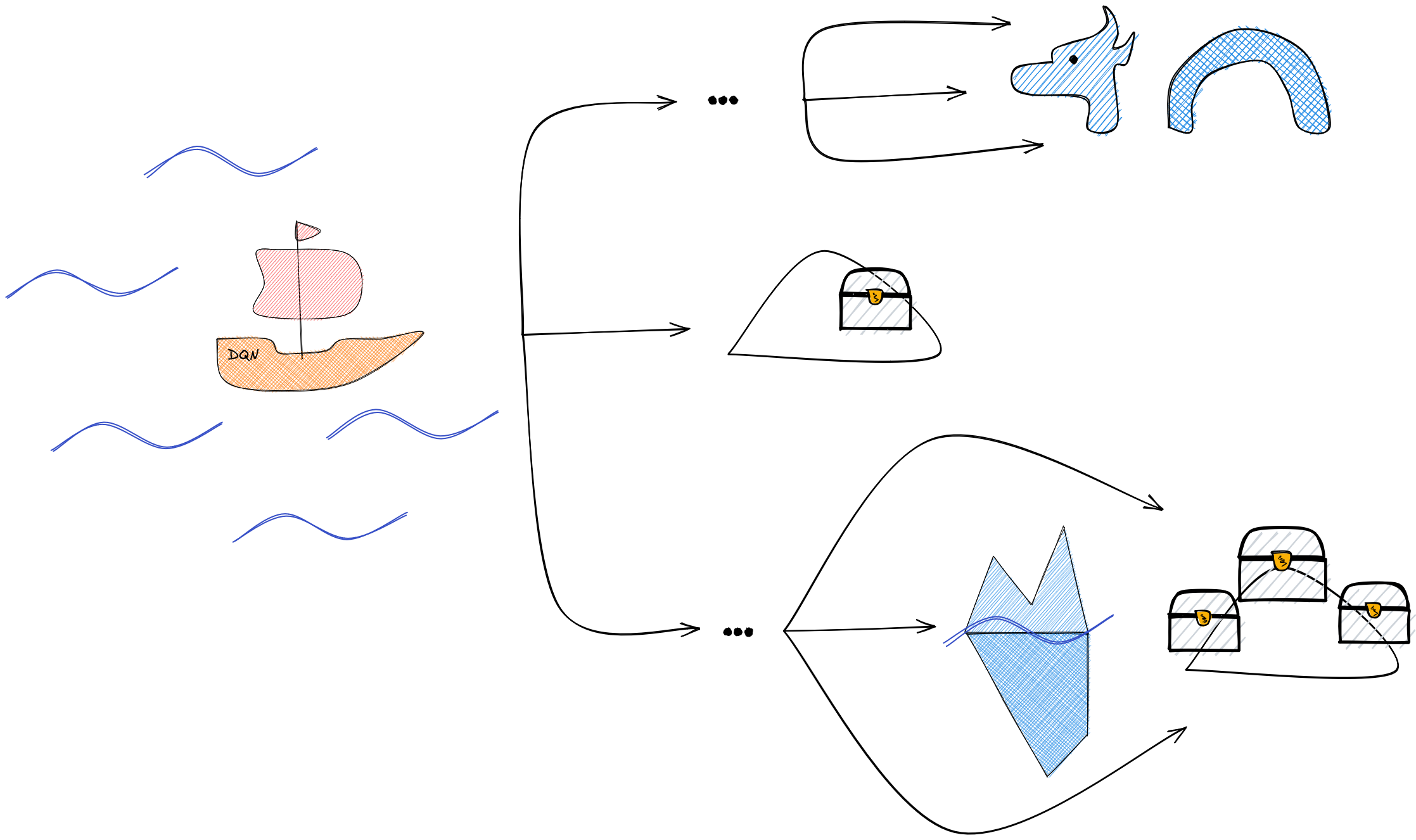#### RL 101 (1/2)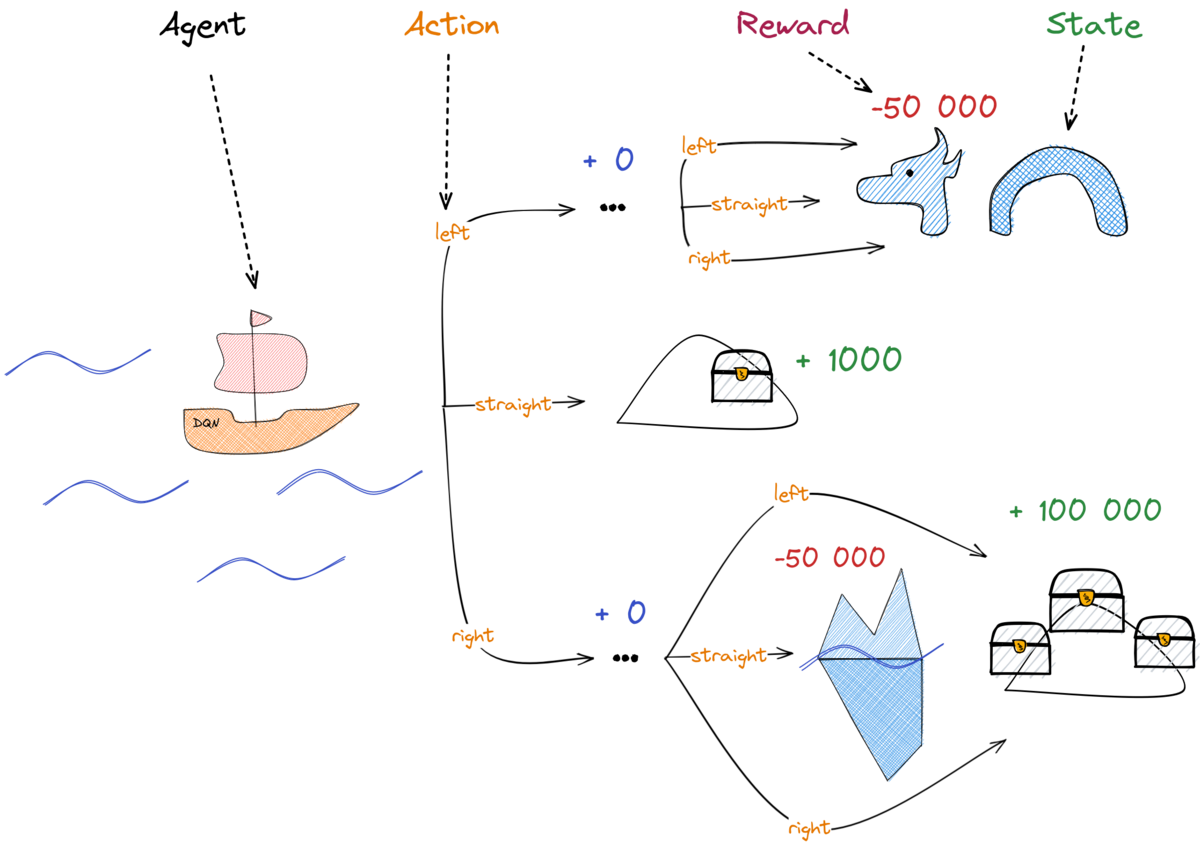#### RL 101 (2/2)

• Agent: the "boat", our main character
• State: Where are we? (position, speed, ...)
• Action: What can we do? (steer left, right, ...)
• Reward: How good are we doing?
• Policy: The "captain", defines the agent's behavior

#### Value Functions

How good is it to be in this state?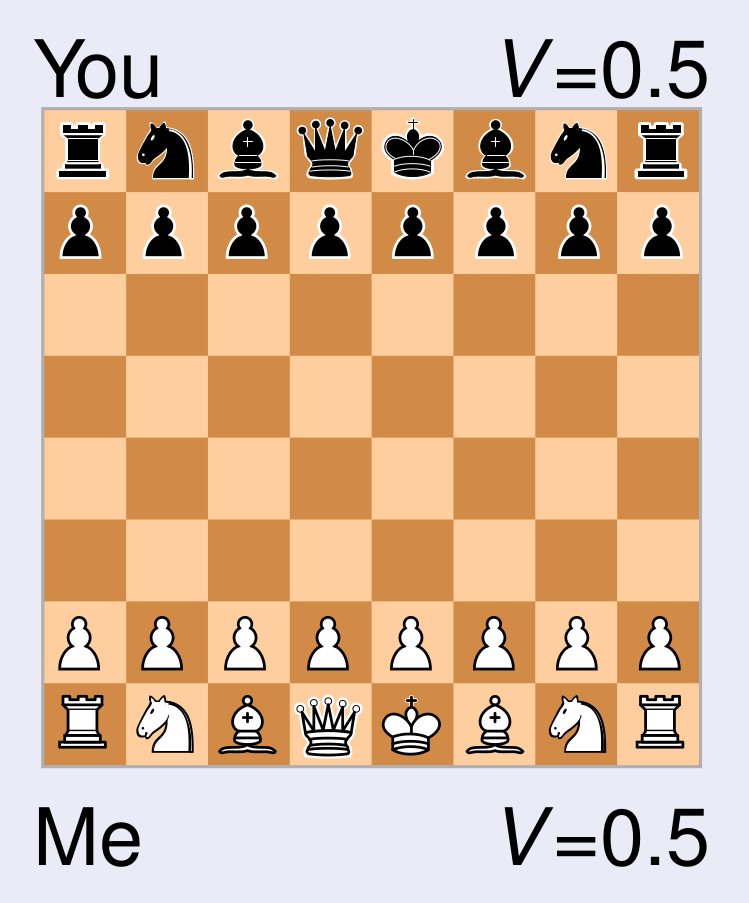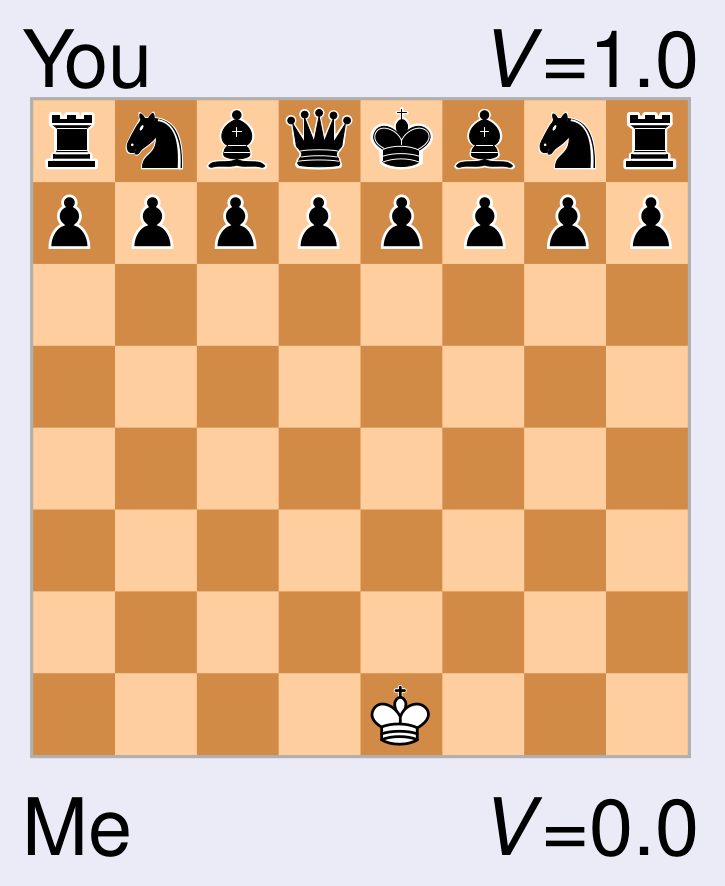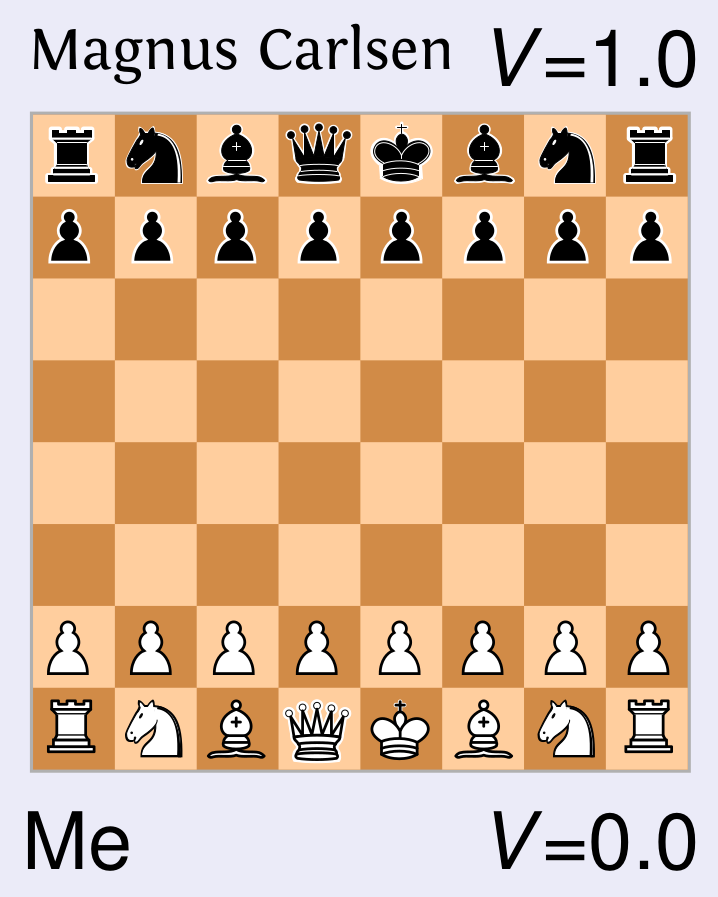Win: 1.0 | Draw: 0.5 | Lose: 0.0

Depends on the state

Depends on the policy

Source: Freek Stulp - Master AIC

##### Action-Value Function: Q-Value

What if we have no model?

Solution: $Q_\pi(s, a)$ instead of $V_\pi(s)$

\begin{aligned} Q_\pi(s, a) = \mathop{\mathbb{E}}[r_t + \gamma r_{t+1} + \gamma^2 r_{t+2} + ... | s_t=s, a_t=a]. \end{aligned}

\begin{aligned} \pi(s) = \argmax_{a \in A} Q_\pi(s, a) \end{aligned}

#### Tabular Q-Learning: Discrete States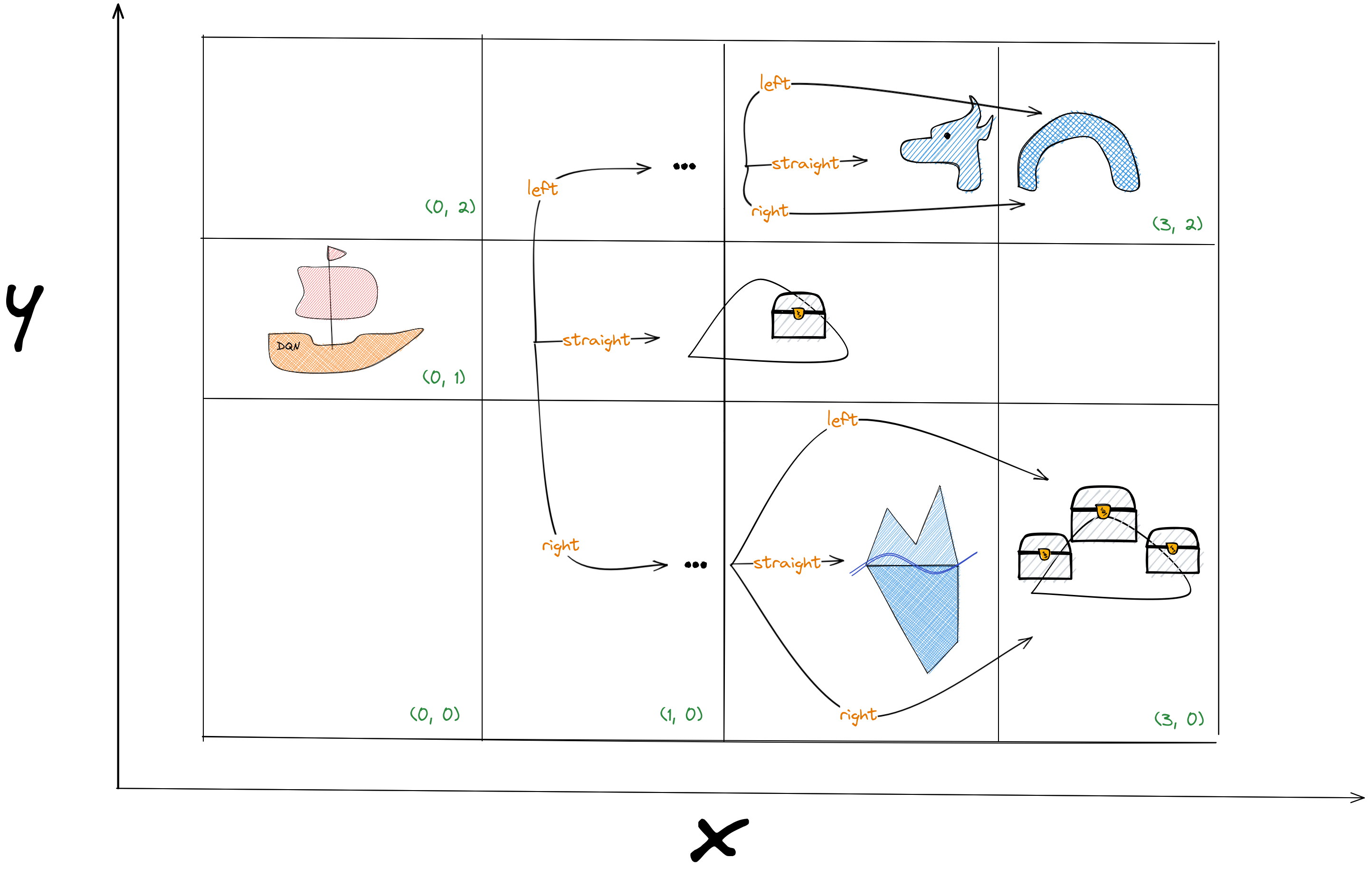#### Tabular Q-Learning: Q-table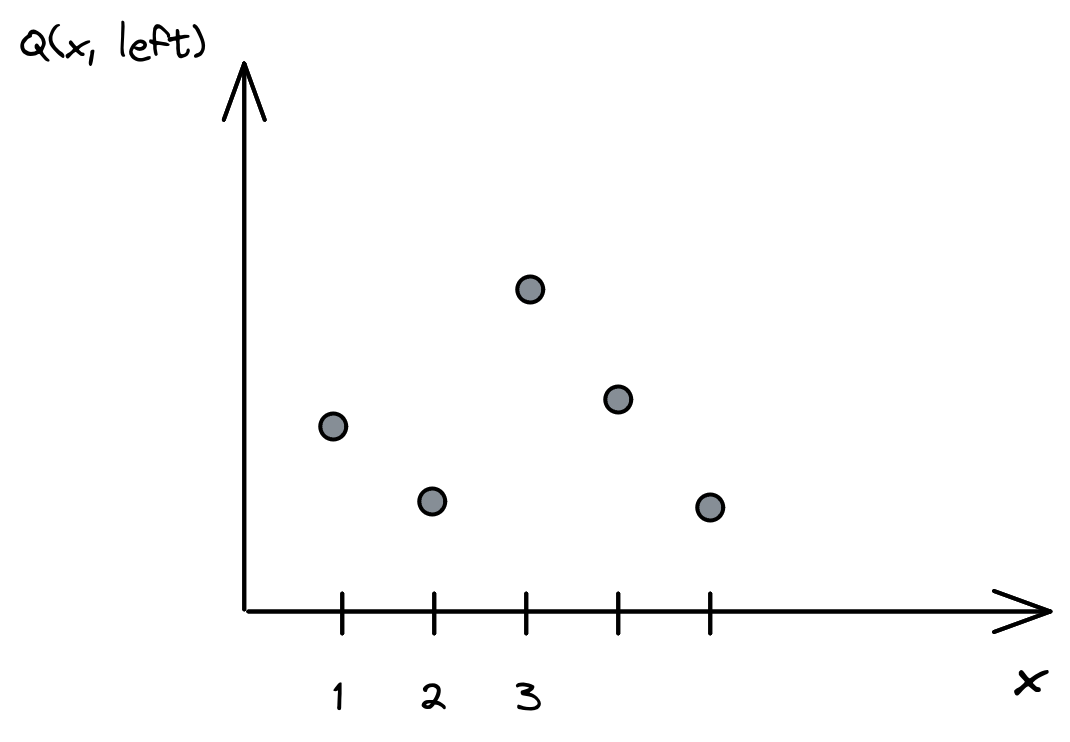#### Tabular Q-Learning: Q-values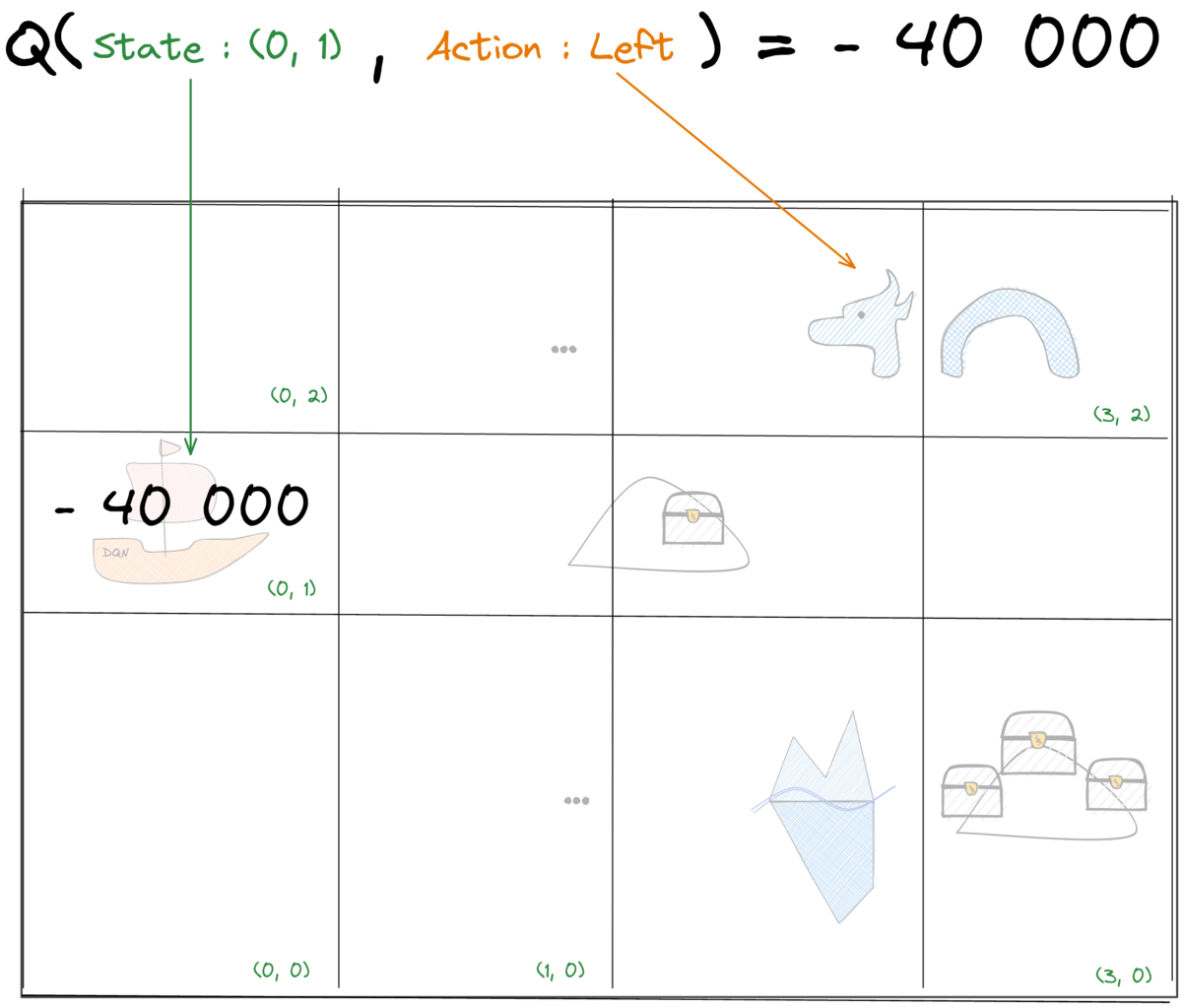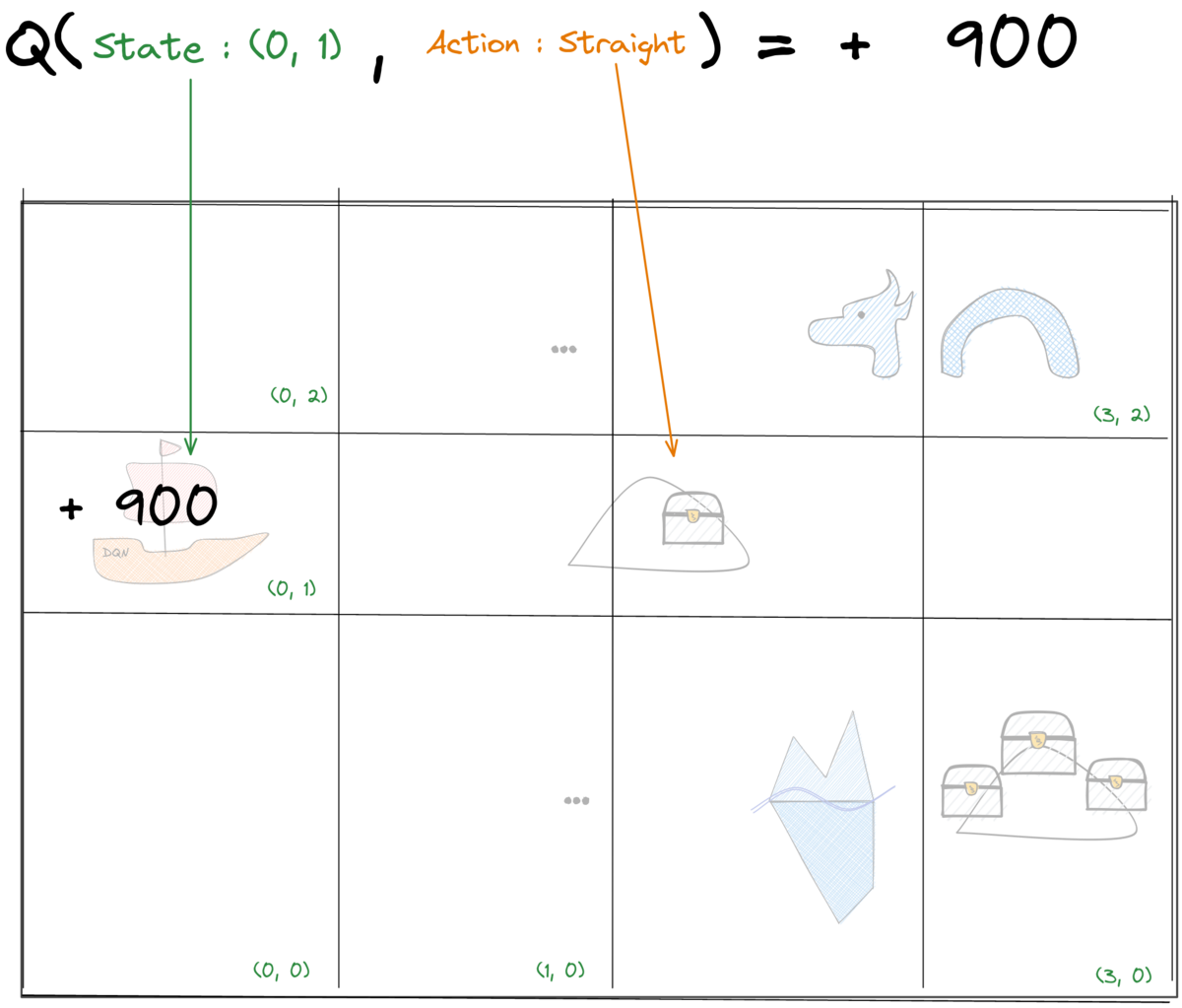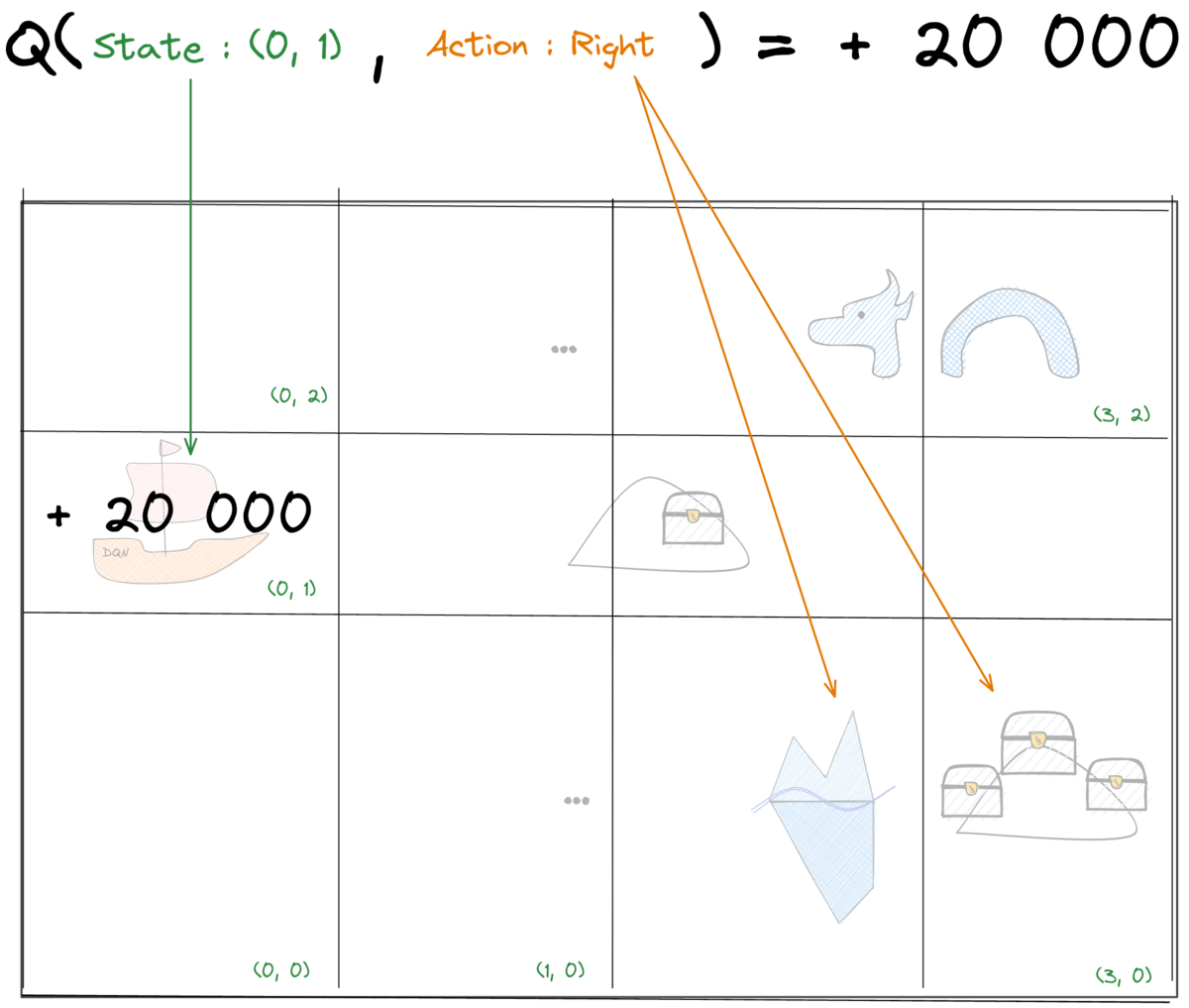#### Tabular Q-Learning: Update rule

Bellman equation for optimal value function:

\begin{aligned} \textcolor{#1864ab}{Q^*(s_t, a_t)} = \mathop{\mathbb{E}}[\textcolor{#a61e4d}{r(s_t, a_t) + \gamma \max_{a'} Q^*(s_{t+1},a')}]. \end{aligned}

Q-learning update rule

\begin{aligned} \textcolor{#1864ab}{Q^n(s_t, a_t)} \gets \textcolor{#1864ab}{Q^{n-1}(s_t, a_t)} + \alpha \cdot (\textcolor{#a61e4d}{r_t + \gamma \cdot \max_{a'} Q^{n-1}(s_{t+1}, a')} - \textcolor{#1864ab}{Q^{n-1}(s_t, a_t)}) \end{aligned}

#### Tabular Q-Learning: Update explained

$\alpha=1$ (learning rate)

\begin{aligned} \textcolor{#1864ab}{Q(s_t, a_t)} = \textcolor{#a61e4d}{r_t + \gamma \cdot \max_{a'} Q(s_{t+1}, a')} \end{aligned}#### Reminder#### Tabular Q-Learning: Terminal State### Questions?

#### Tabular Q-Learning: Limitations

• Discrete states
• No generalization (lookup table)
• Discrete actions

#### How to go beyond tabular Q-Learning?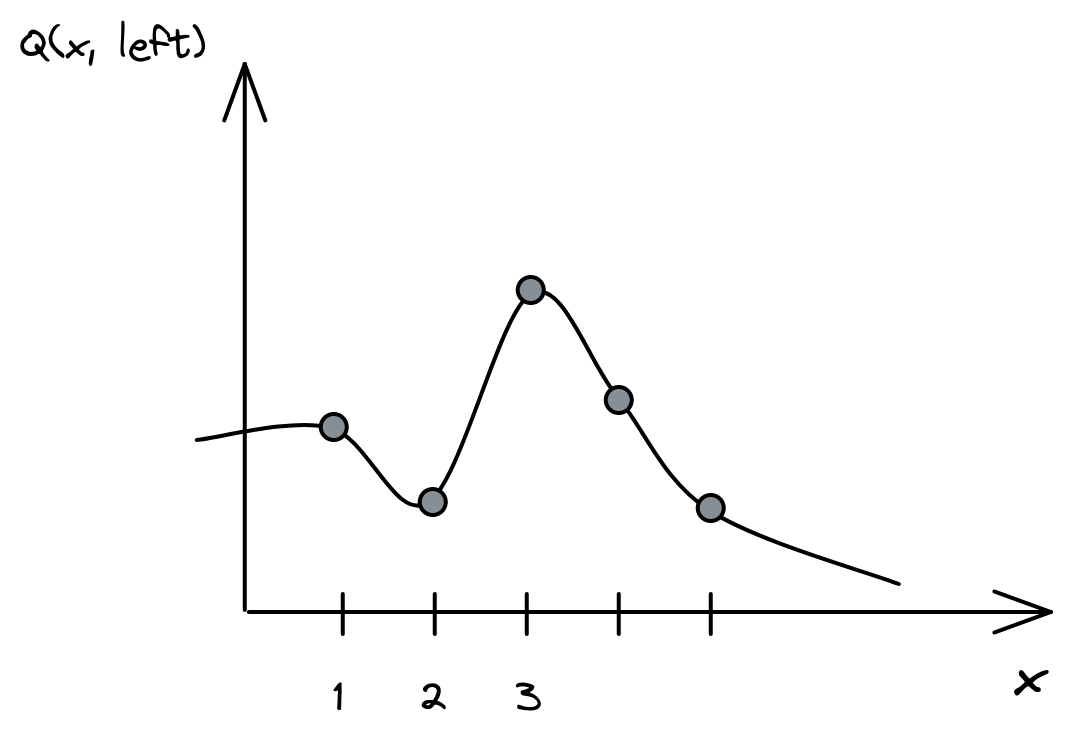### Q-Value Estimator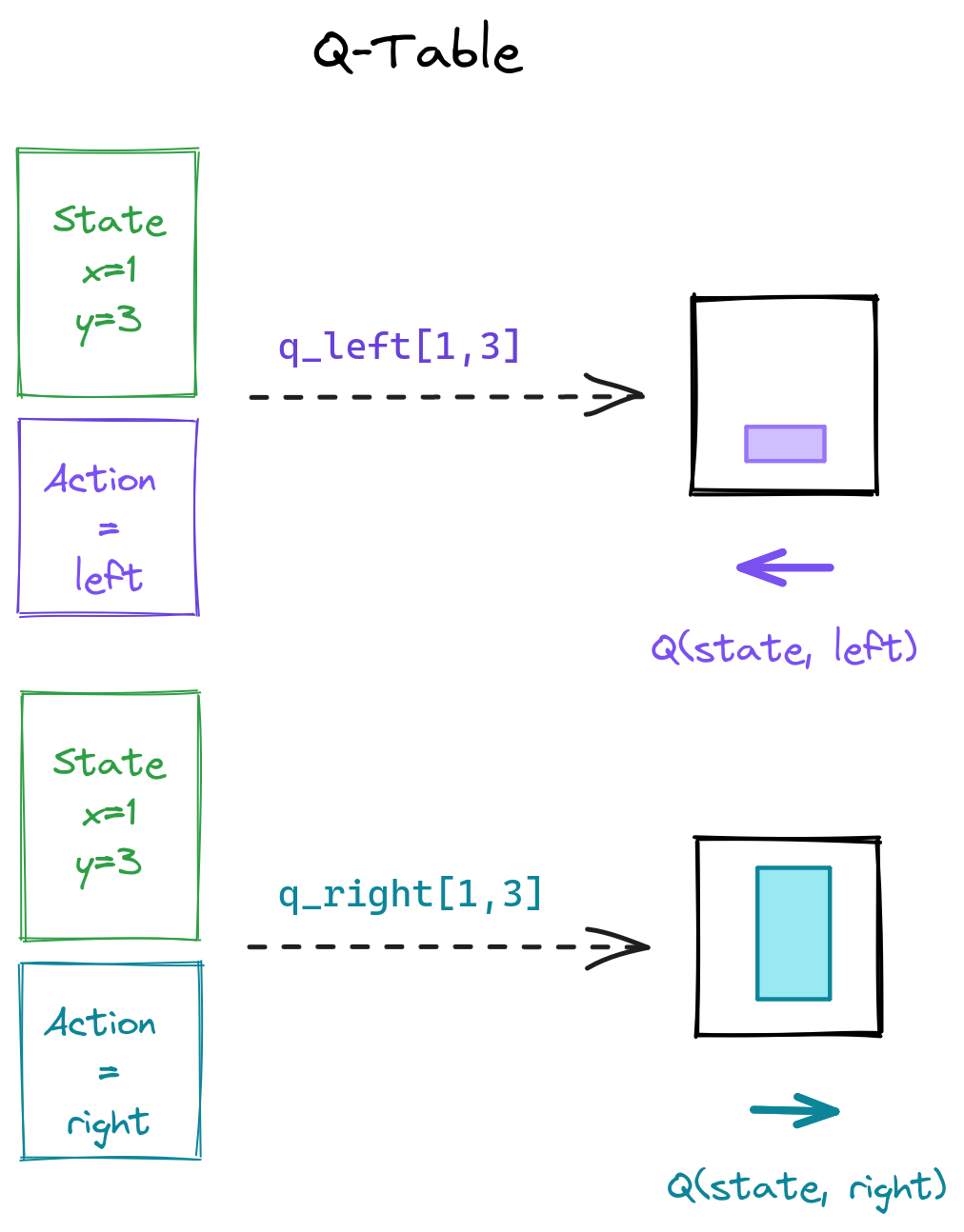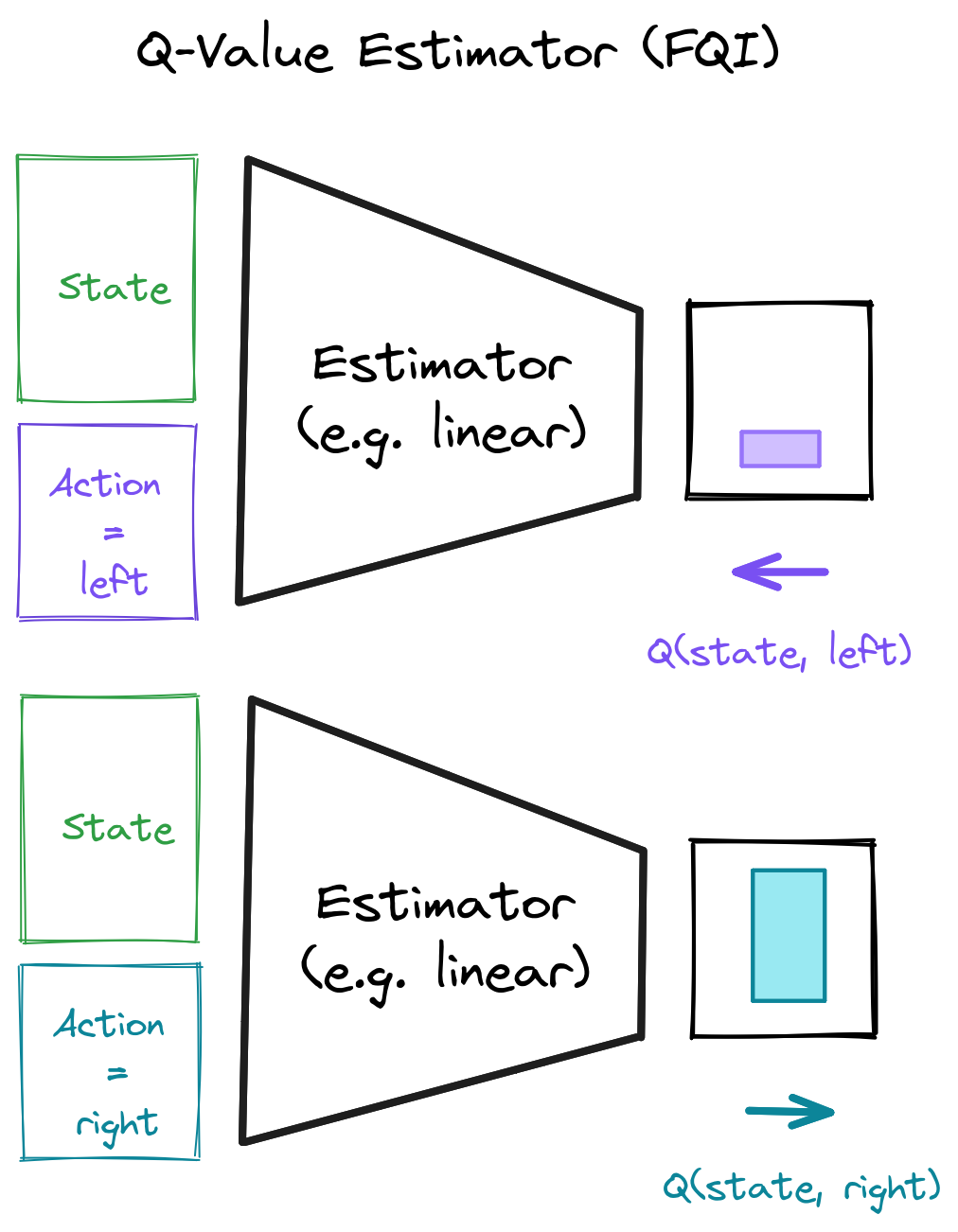#### Q-Learning Regression (1/2)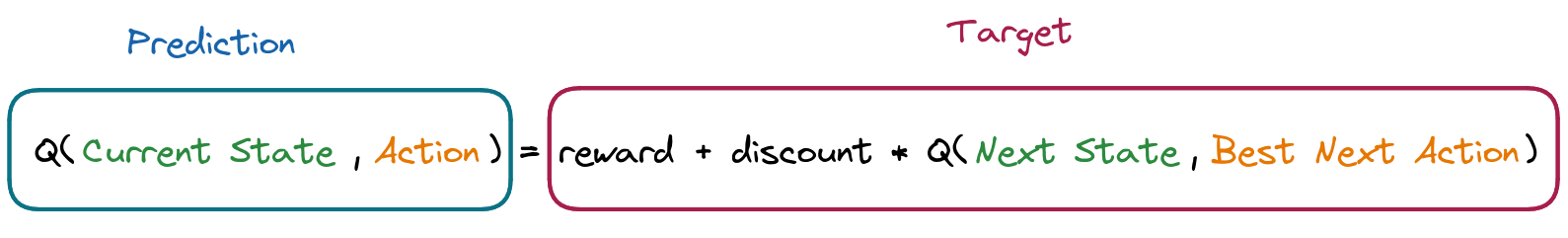\begin{aligned} \textcolor{#1864ab}{Q_{\textcolor{black}{\theta}}(s_t, a_t)} = \textcolor{#a61e4d}{r_t + \gamma \cdot \max_{a' \in A}(Q_{\textcolor{black}{\theta}}(s_{t+1}, a'))} \end{aligned}
\begin{aligned} \textcolor{#1864ab}{f_{\textcolor{black}{\theta}}(x)} = \textcolor{#a61e4d}{y} \end{aligned}
$\theta$: parameters of the estimator

#### One small detail...

$\textcolor{#1864ab}{Q_\theta(s_t, a_t)}$ depends on $\textcolor{#a61e4d}{Q_\theta(s_{t+1}, a')}$...

What can we do about it?

Iterate!     Use $Q^{\textcolor{green}{n}}_\theta(s_t, a_t)$

#### Fitted Q-Iteration (FQI) (1/2)

1. Create the training set based on the previous iteration $Q^{\textcolor{green}{n-1}}_\theta(s, a)$ and the transitions:
• input: $\textcolor{#1864ab}{x = (s_t, a_t)}$
• if $s_{t+1}$ is non terminal:    $y = r_t + \gamma \cdot \max_{a' \in A}(Q^{n-1}_\theta(s_{t+1}, a'))$
• if $s_{t+1}$ is terminal:             $\textcolor{a61e4d}{y = r_t}$
2. Fit a model using a regression algorithm to obtain $Q^{\textcolor{green}{n}}_\theta(s, a)$
\begin{aligned} \textcolor{#1864ab}{f_\theta(x)} = \textcolor{#a61e4d}{y} \end{aligned}
3. Repeat, $\textcolor{green}{n = n + 1}$

#### Fitted Q-Iteration (2/2)

For $\textcolor{green}{n = 0}$, the initial training set is defined as:
• $\textcolor{#1864ab}{x = (s_t, a_t)}$
• $\textcolor{#a61e4d}{y = r_t}$

#### Fitted Q-Iteration (code)


initial_targets = rewards
# Initial Q-value estimate
qf_input = np.concatenate((states, actions))
qf_model.fit(qf_input, initial_targets)

for _ in range(N_ITERATIONS):
# Re-use Q-value model from previous iteration
# to create the next targets
next_q_values = get_max_q_values(qf_model, next_states)
# Non-terminal states target
targets[non_terminal_states] = rewards + gamma * next_q_values
# Special case for terminal states
targets[terminal_states] = rewards
# Update Q-value estimate
qf_model.fit(qf_input, targets)


### CartPole Env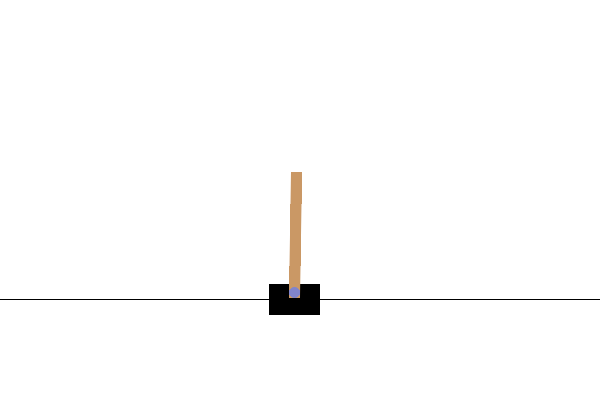### Gym/Gymnasium API


import gymnasium as gym

# Create the environment
env = gym.make("CartPole-v1", render_mode="human")
# Reset env and get first observation
obs, _ = env.reset()

# Step in the env with random actions and display the env
for _ in range(100):
env.render() # Display the env

action = env.action_space.sample()
# Retrieve new observation, reward,
# termination signal, truncation signal
next_obs, reward, terminated, truncated, info = env.step(action)
# Update current observation
obs = next_obs
# End of an episode
if terminated or truncated:
obs, _ = env.reset()



### FQI Limitations

• Offline RL
• Loop over all possible actions $A$ to get next best action $\textcolor{#a61e4d}{a'}$: \begin{aligned} \max_{\textcolor{#a61e4d}{a' \in A}} Q_\theta(s_{t+1}, \textcolor{#a61e4d}{a'}) \end{aligned}
• Instability (target depends on $Q^{n-1}_\theta(s_{t+1}, a')$)

### From FQI to DQN

• Offline RL Online RL
• Loop over actions One forward pass to get all $Q_\theta(s, a)$
• Instability Target Network $Q_{\textcolor{green}{\theta'}}(s, a)$

### Deep Q-Network (DQN)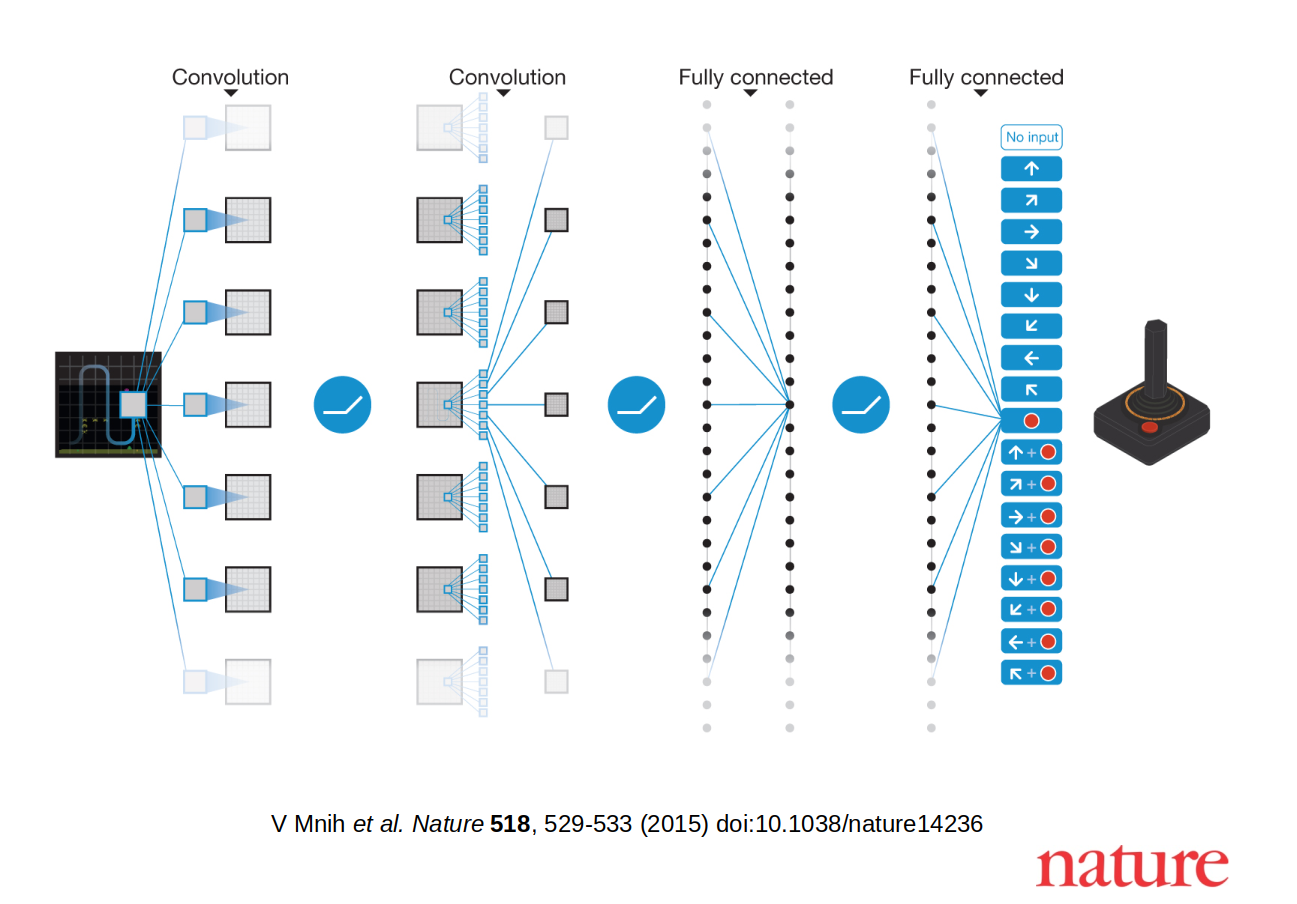### Replay Buffer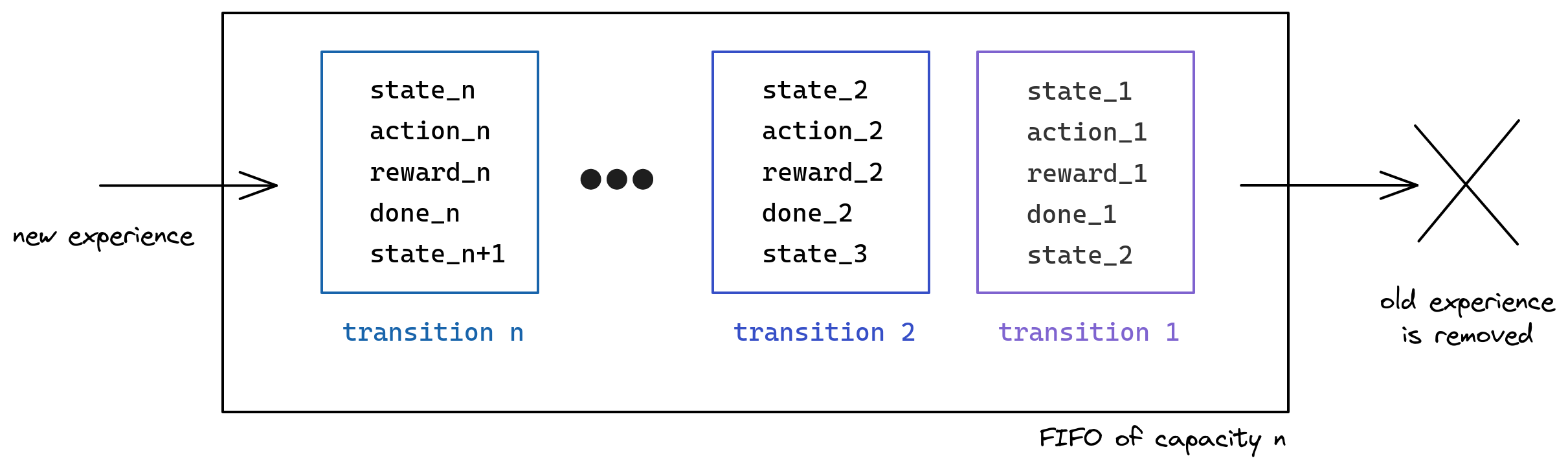### Replay Buffer Sampling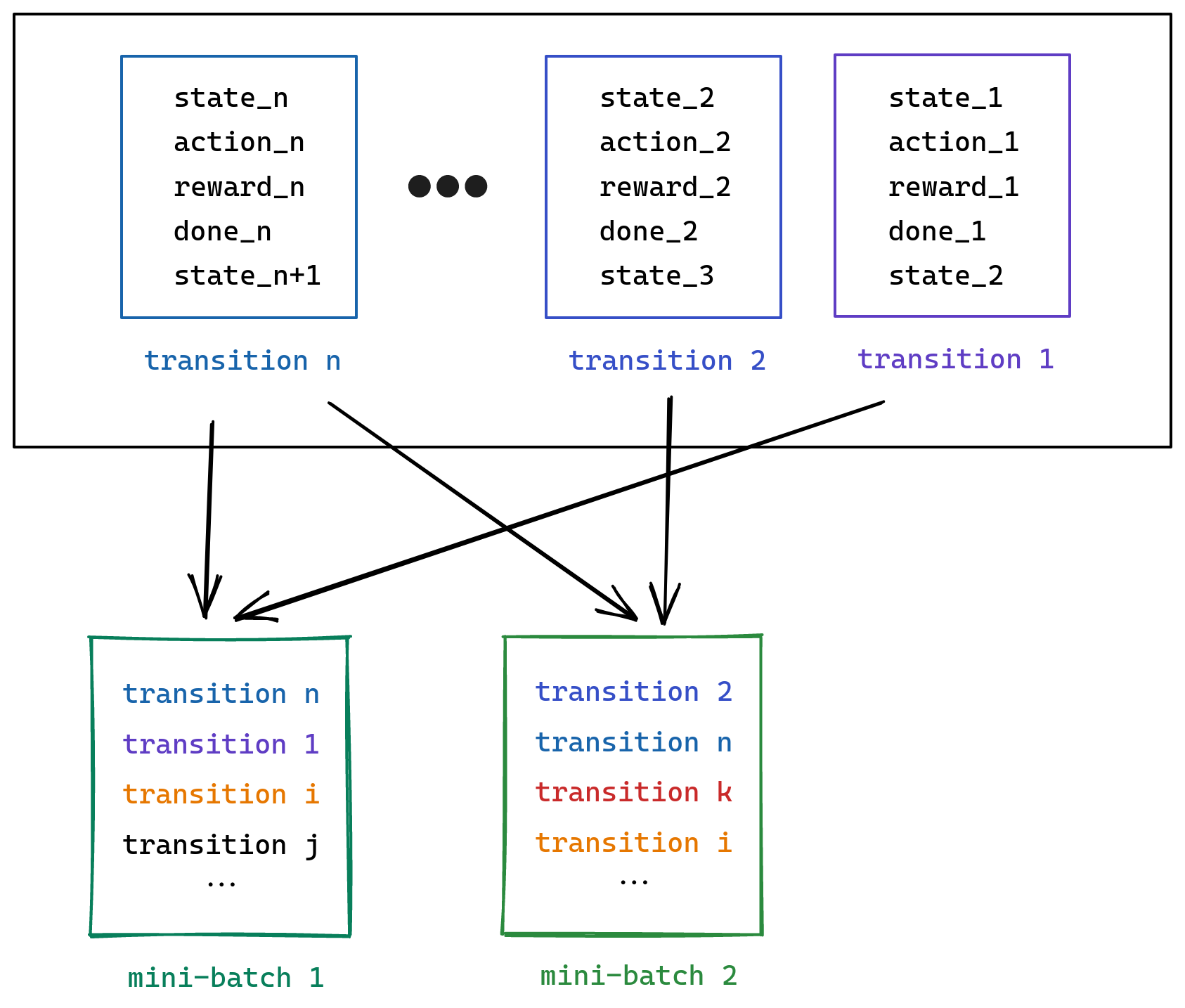### Q-network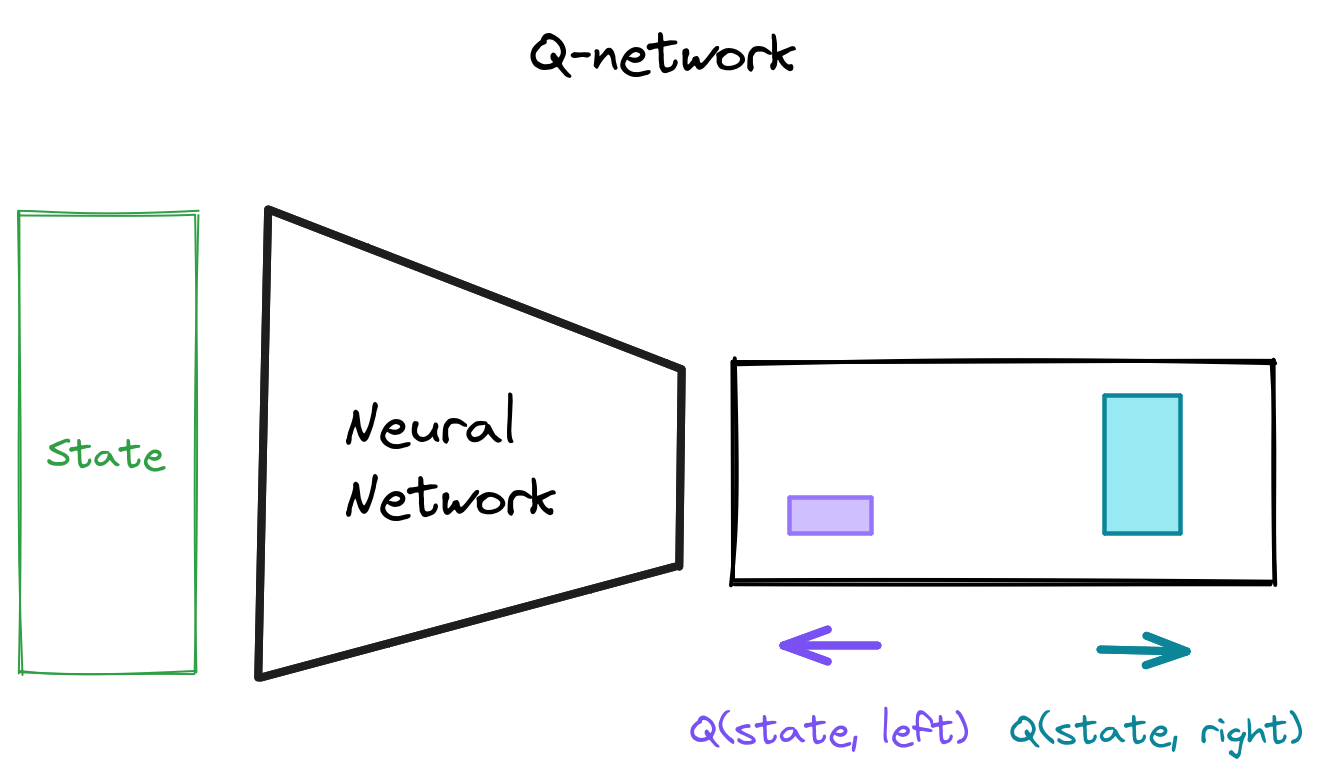### The training loop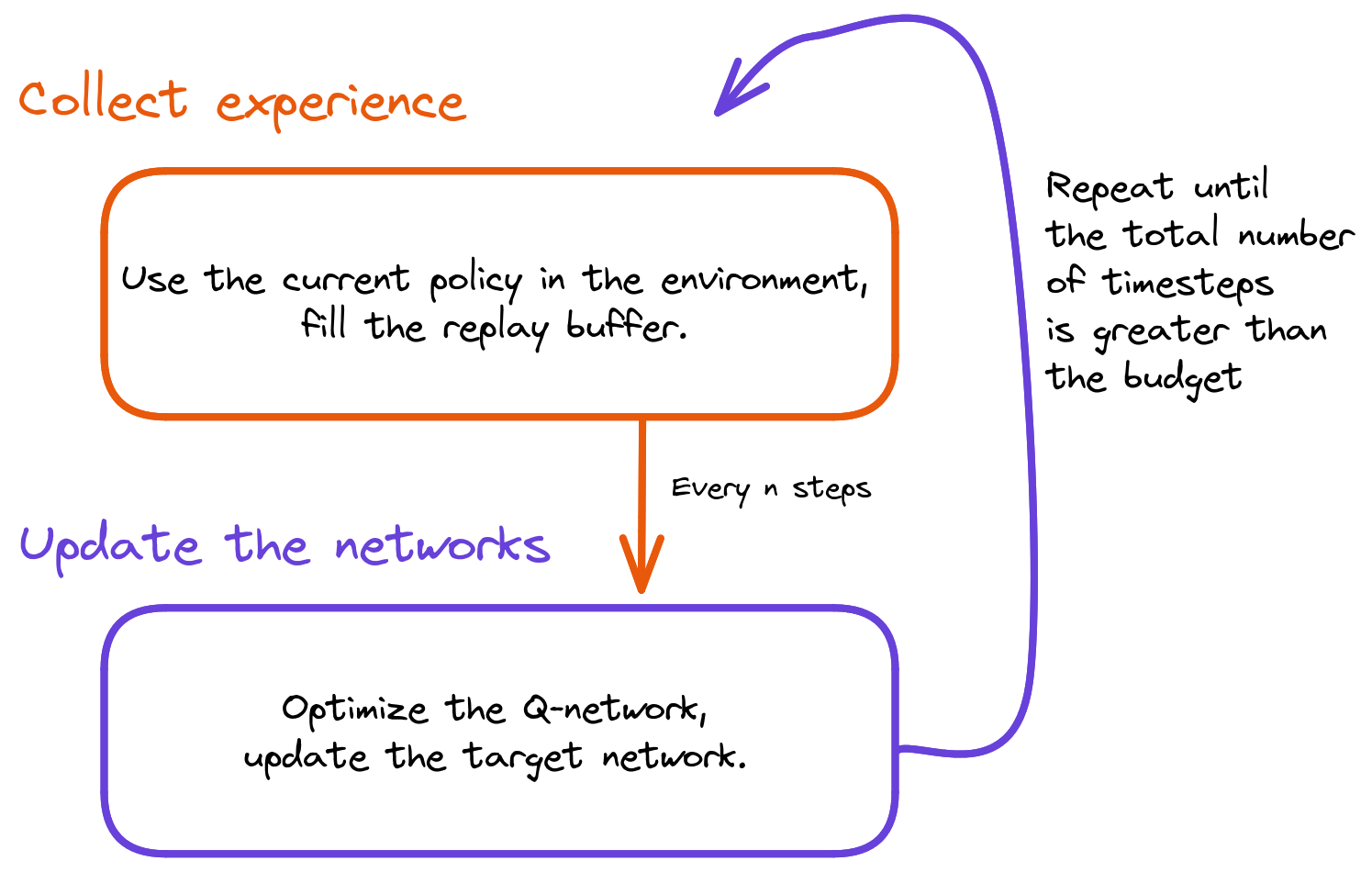### Collecting Experience


# Retrieve q values for the current observation
q_values = q_model(current_obs)

# take the action with the highest q_value
action = np.argmax(q_values)

# Do one step in the env
next_obs, reward, terminated, _, _ = env.step(action)

# Store transition in the replay buffer
replay_buffer.store(obs, action, reward, terminated, next_obs)


### Exploration / Exploitation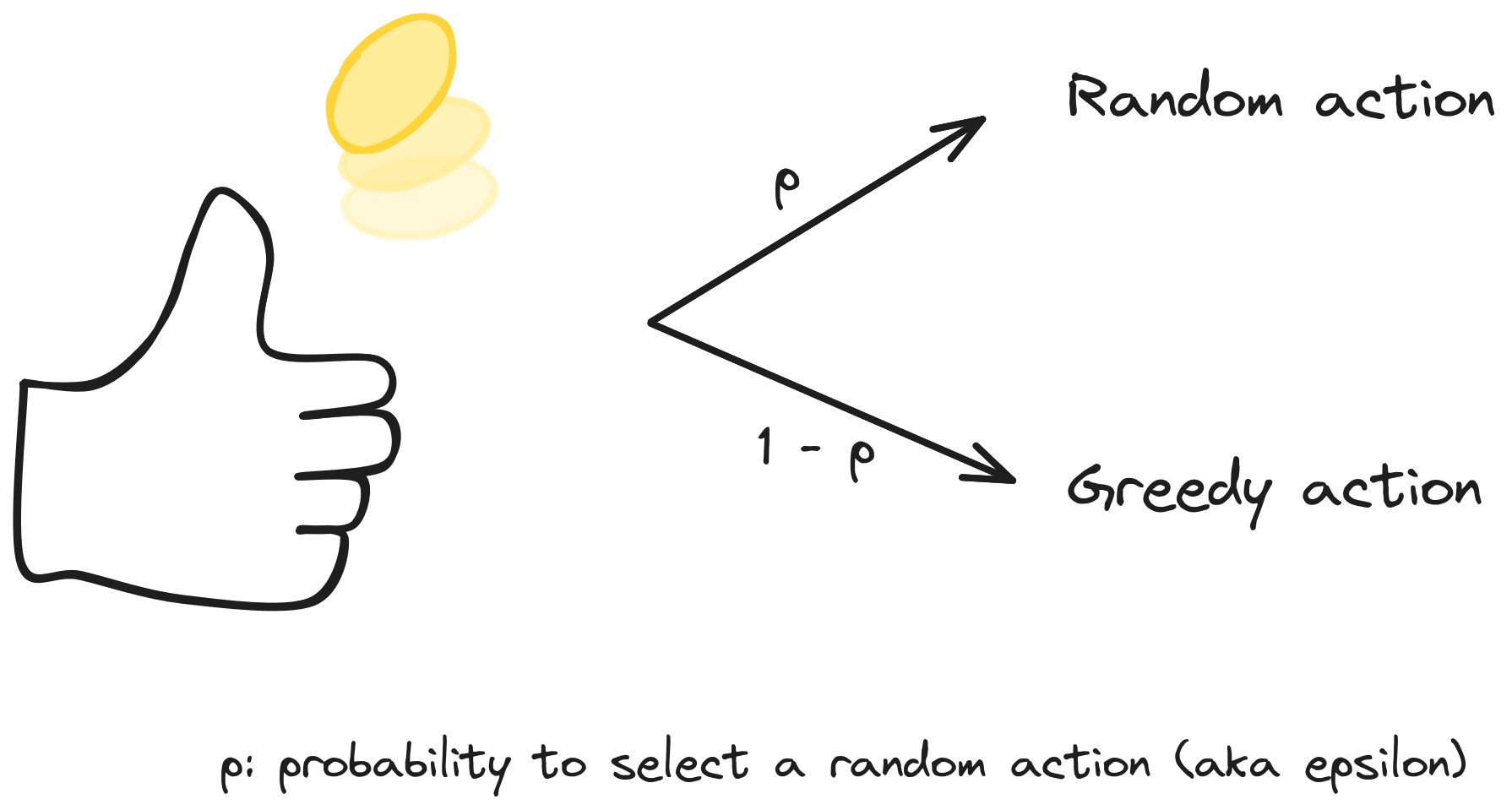### Epsilon-greedy Exploration


# Flip a biased coin
take_random_action = np.random.rand() < exploration_rate

if take_random_action:
# Random action
action = action_space.sample()
else:
# Greedy action
action = np.argmax(q_values)


### Exploration Schedule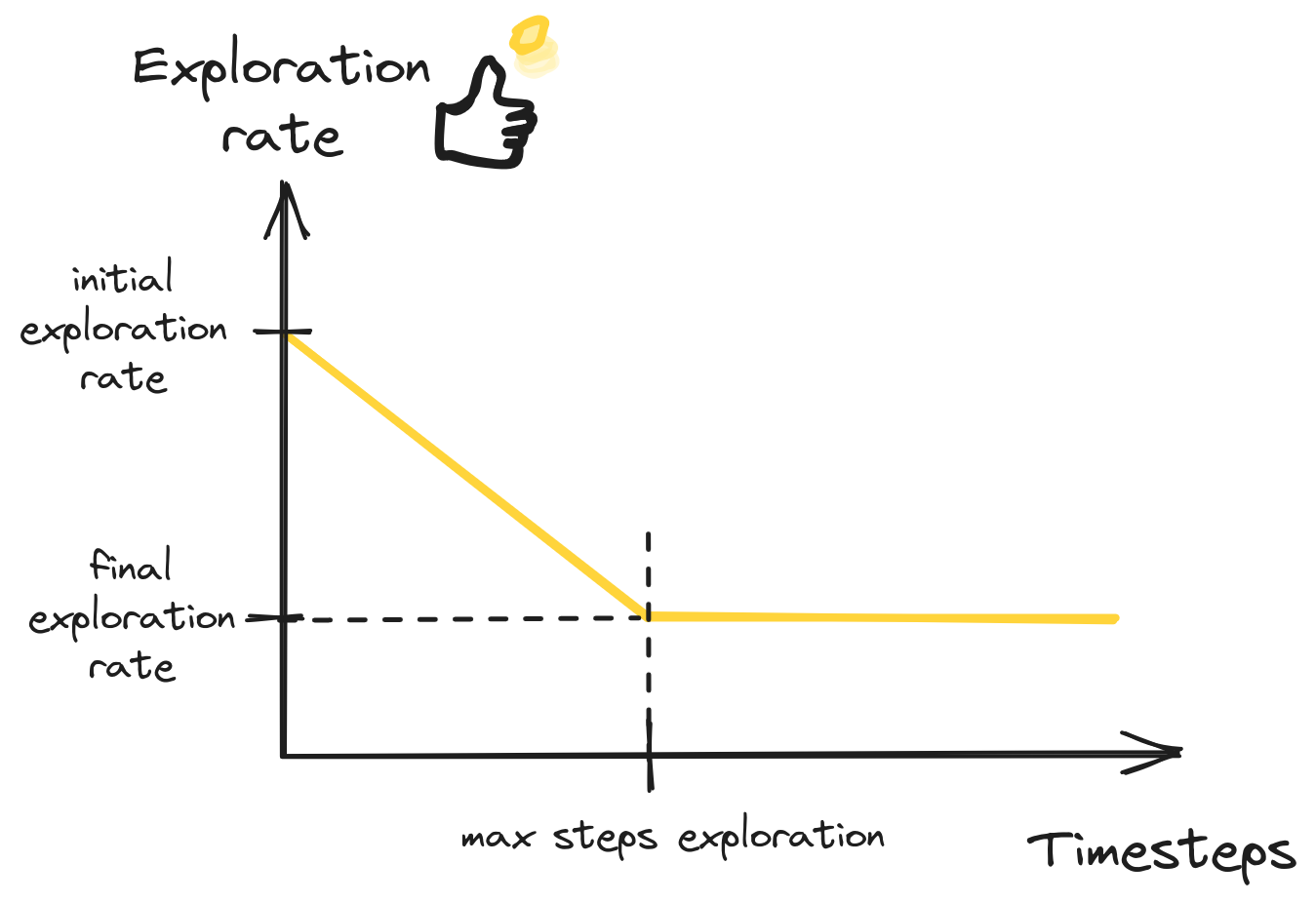### Annotated DQN Algorithm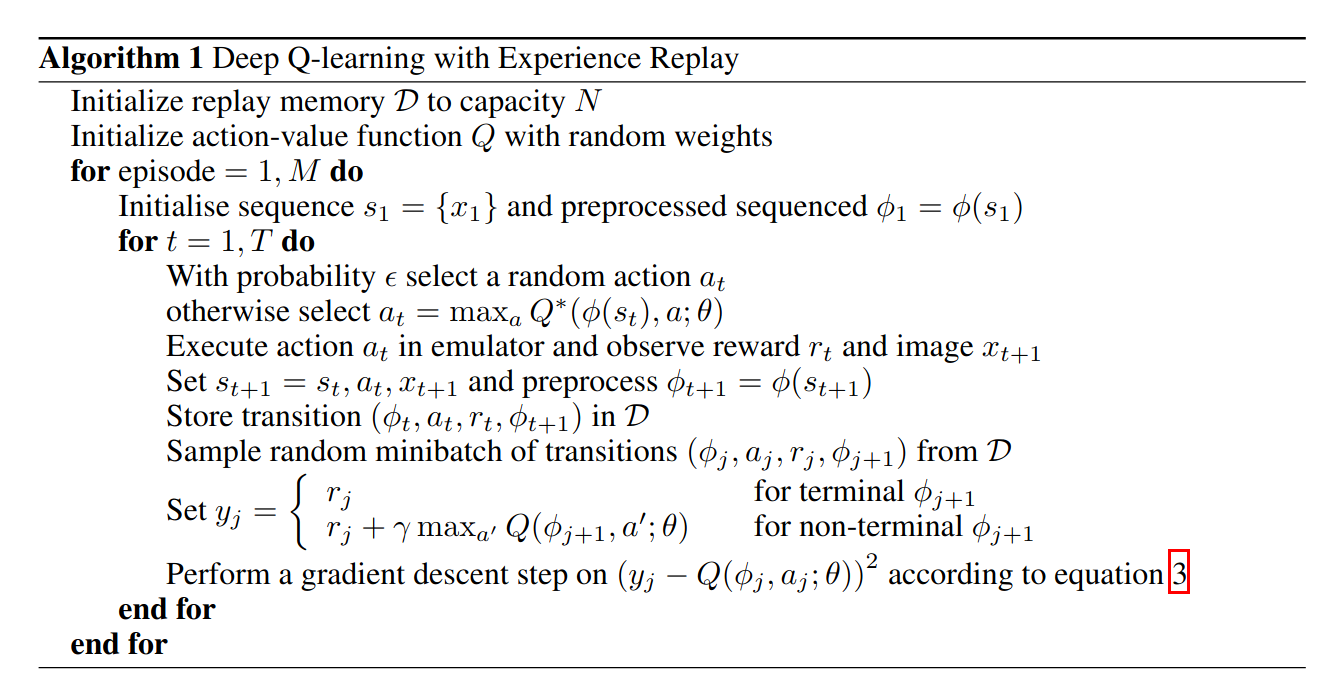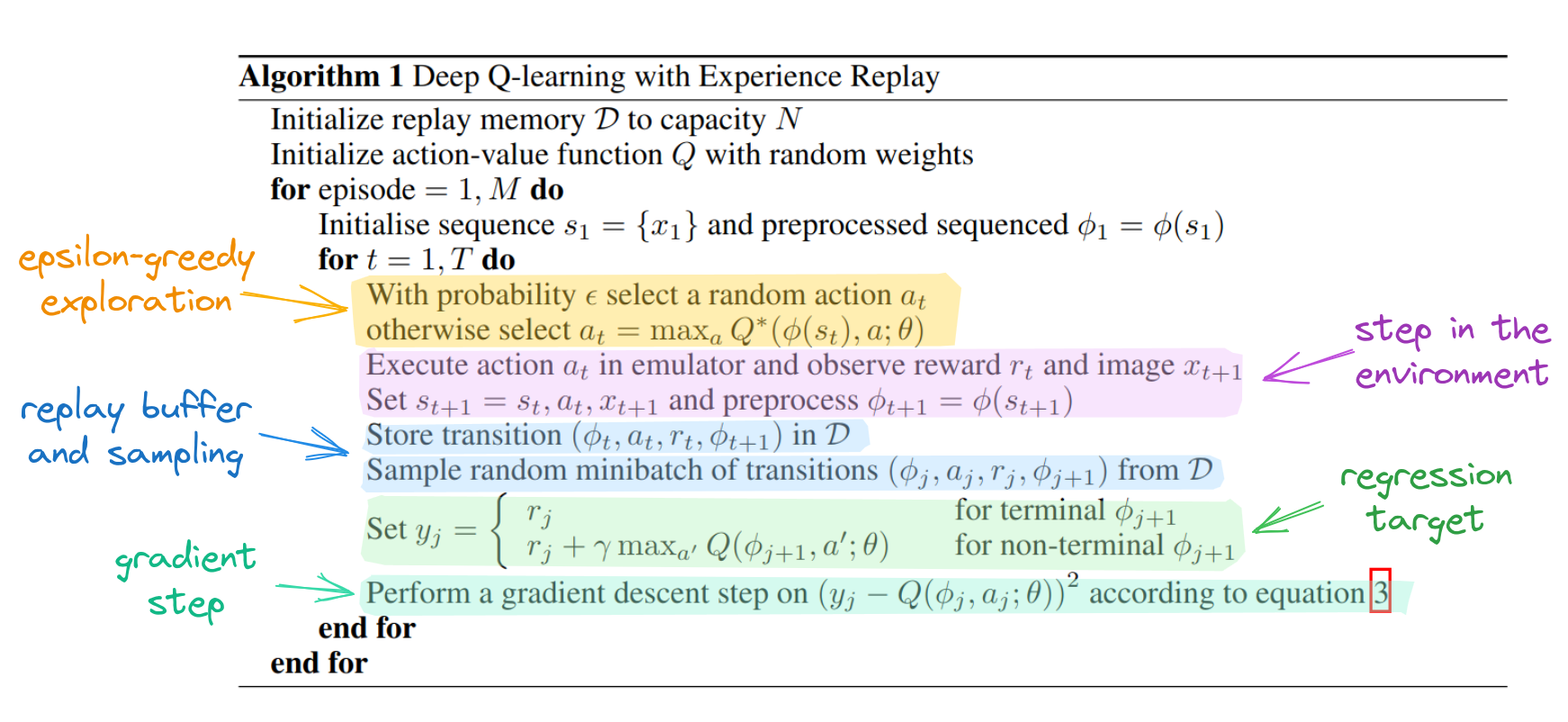### Target Q-Network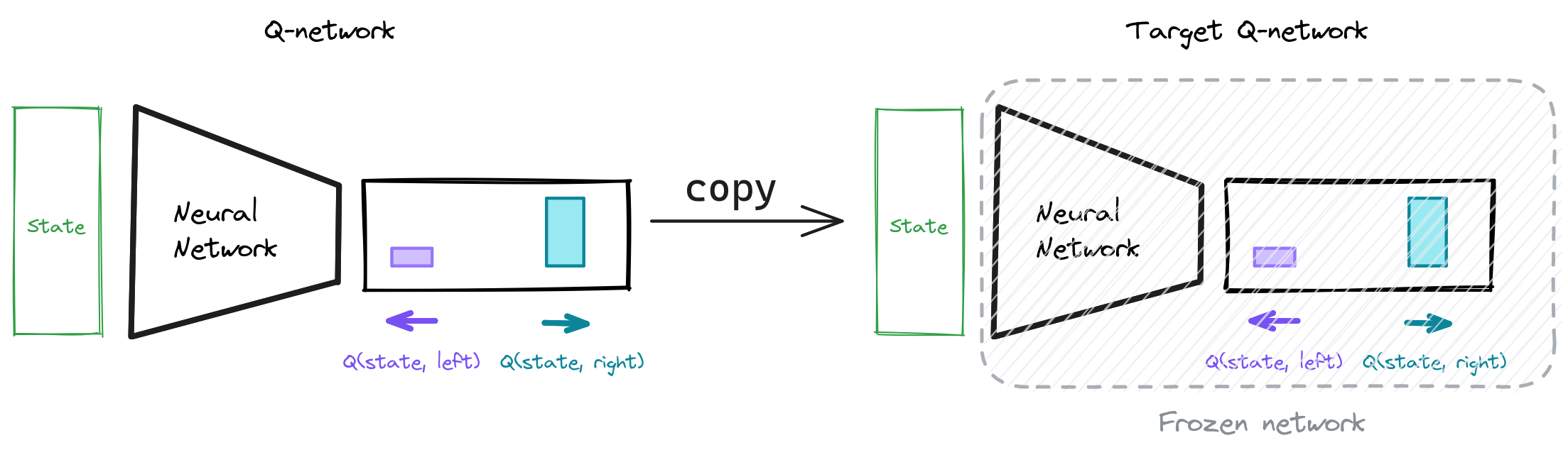### DQN Overview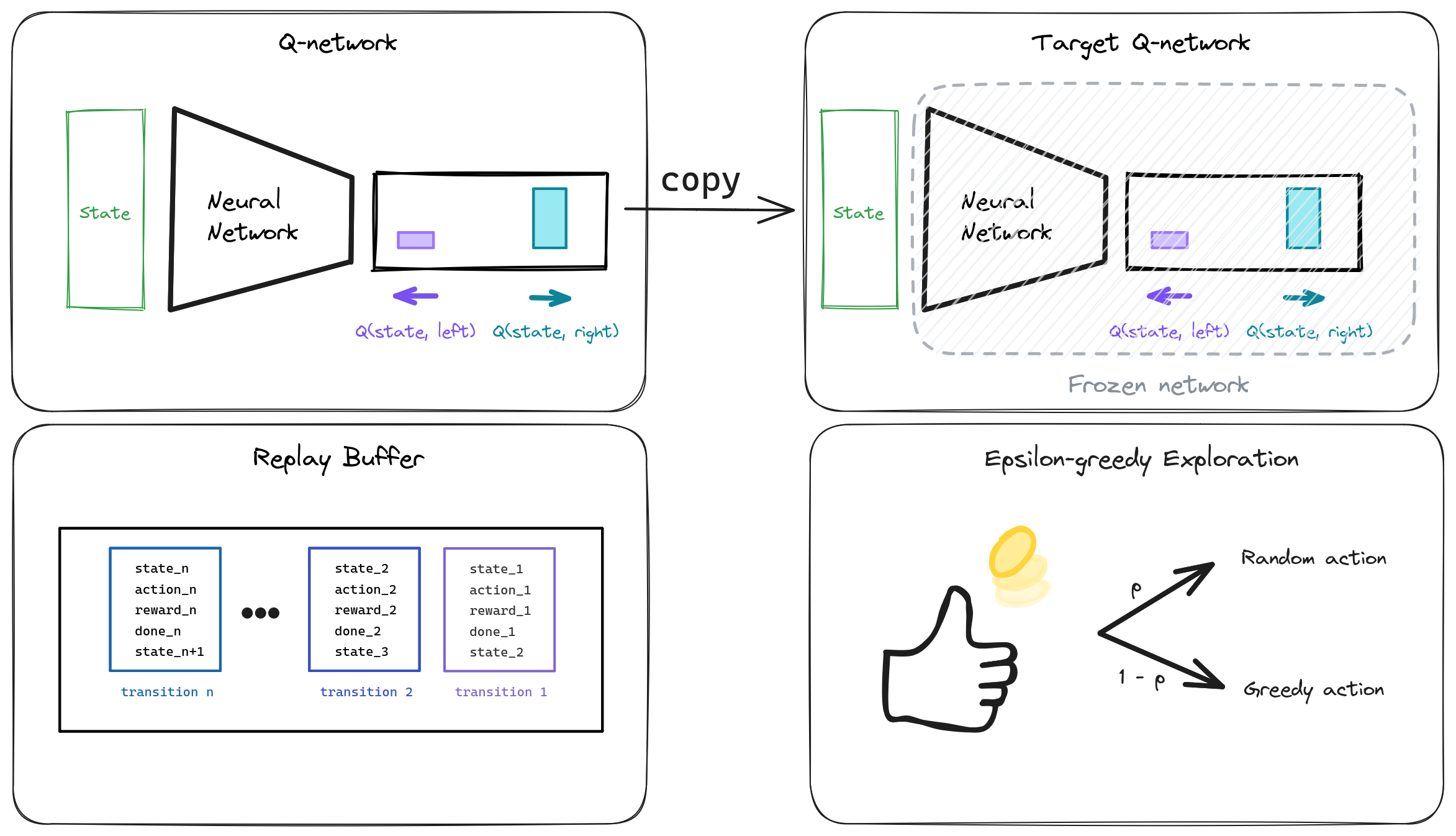### PyTorch API

NumPy PyTorch
np.array([[1, 2], [3, 4]]) th.tensor([[1, 2], [3, 4]])
np.ones((2, 3)) th.ones(2, 3)
np.concatenate th.cat
x.shape x.shape
x.argmax(axis=...) x.argmax(dim=...)
x.item() x.item()
NumPy to PyTorch: th.as_tensor

## Backup Slides

### Regression 101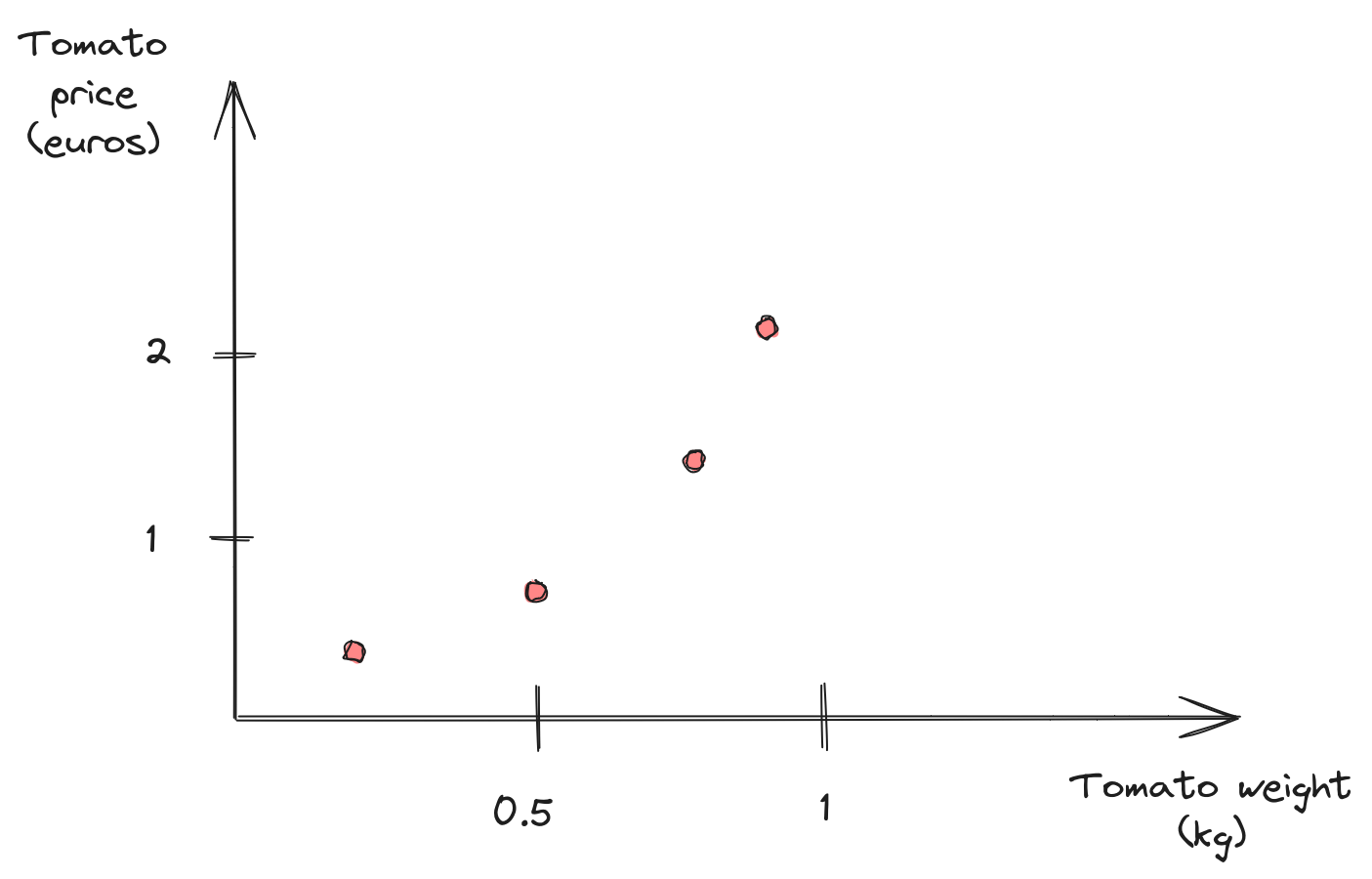#### Linear model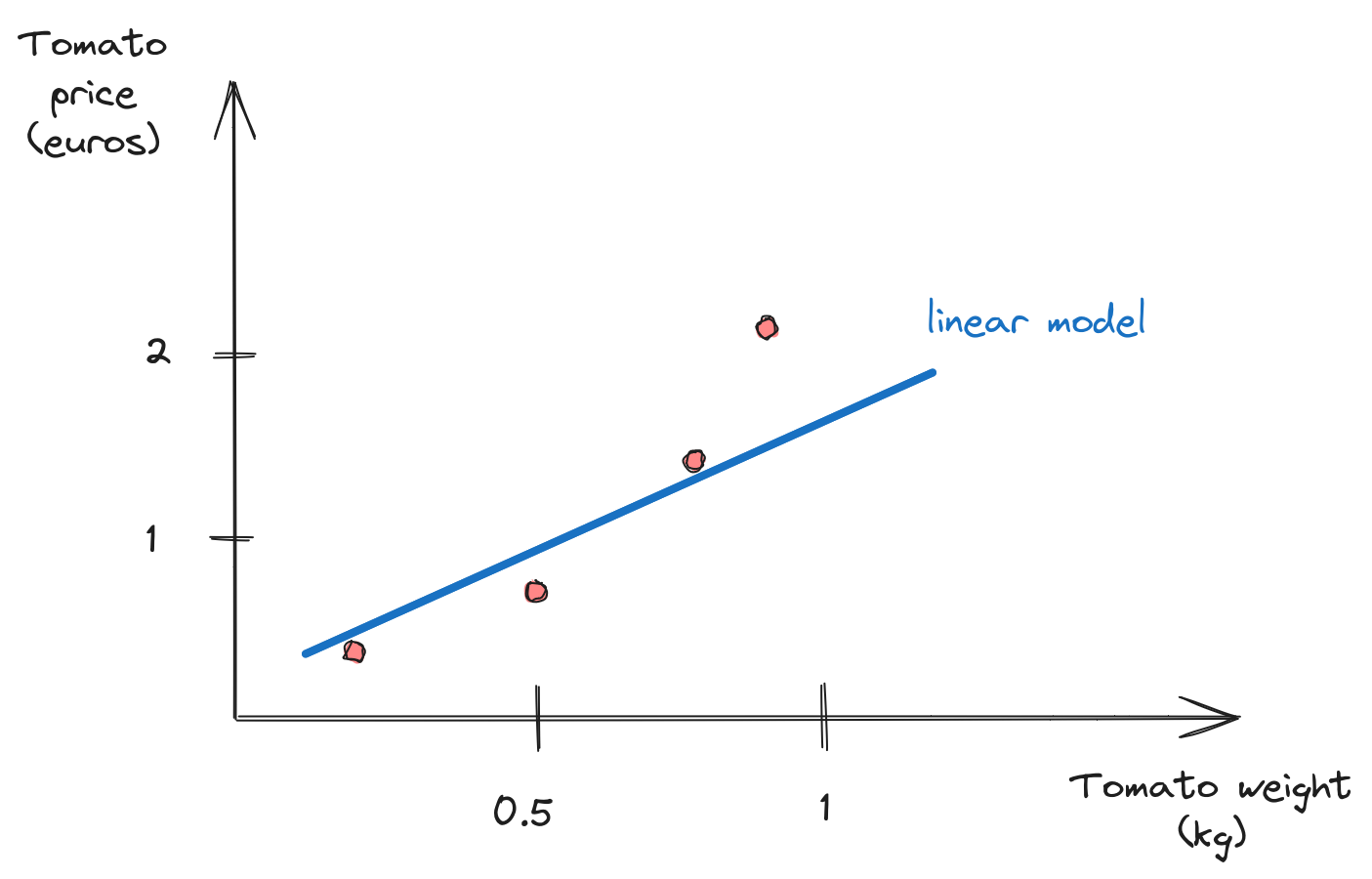#### Linear model(s)?

\begin{aligned} \textcolor{#a61e4d}{y} = \textcolor{#1864ab}{f_\theta(x)} \quad ; \quad \theta = \{\text{slope}, \text{bias}\} \end{aligned}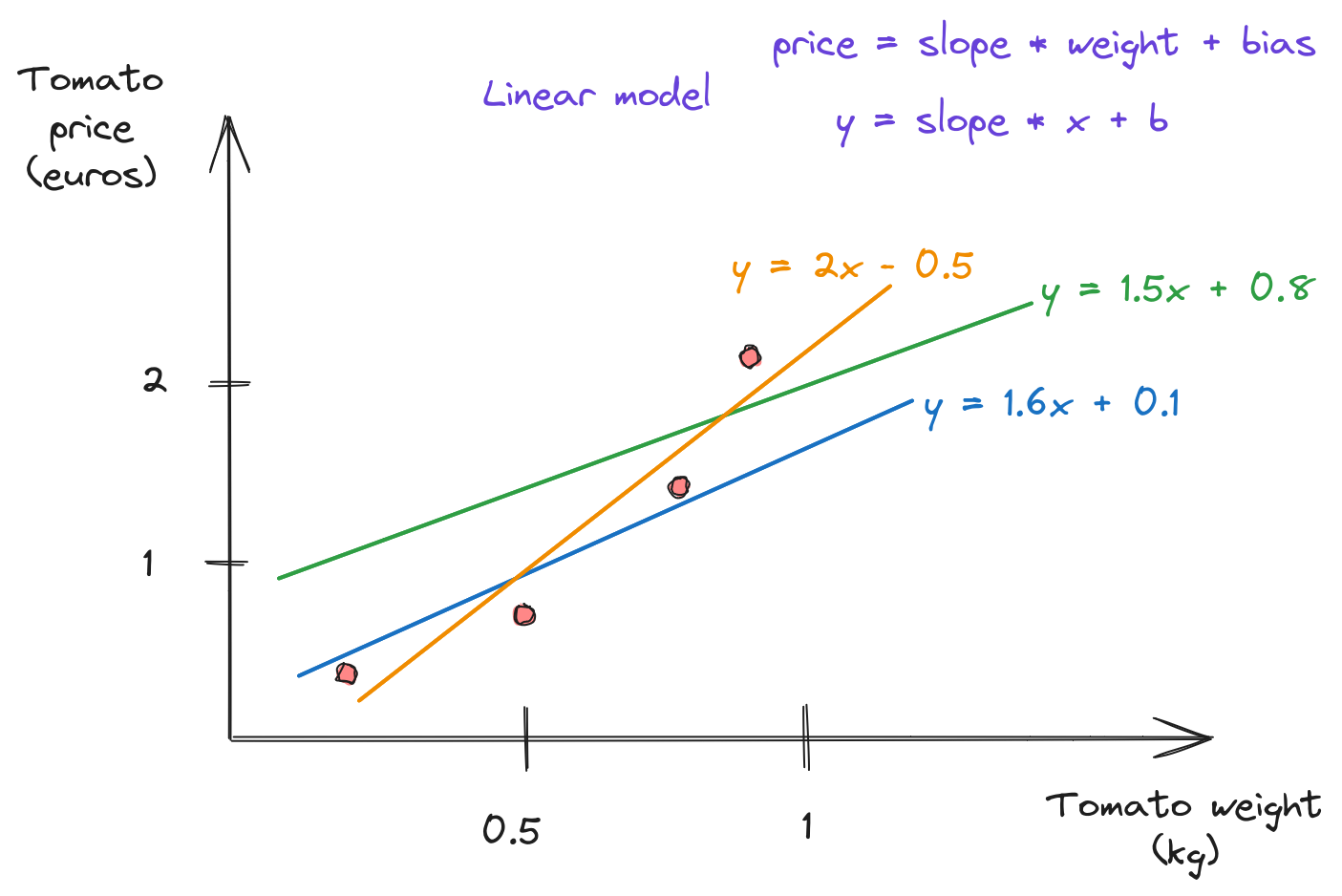#### Non Linear Model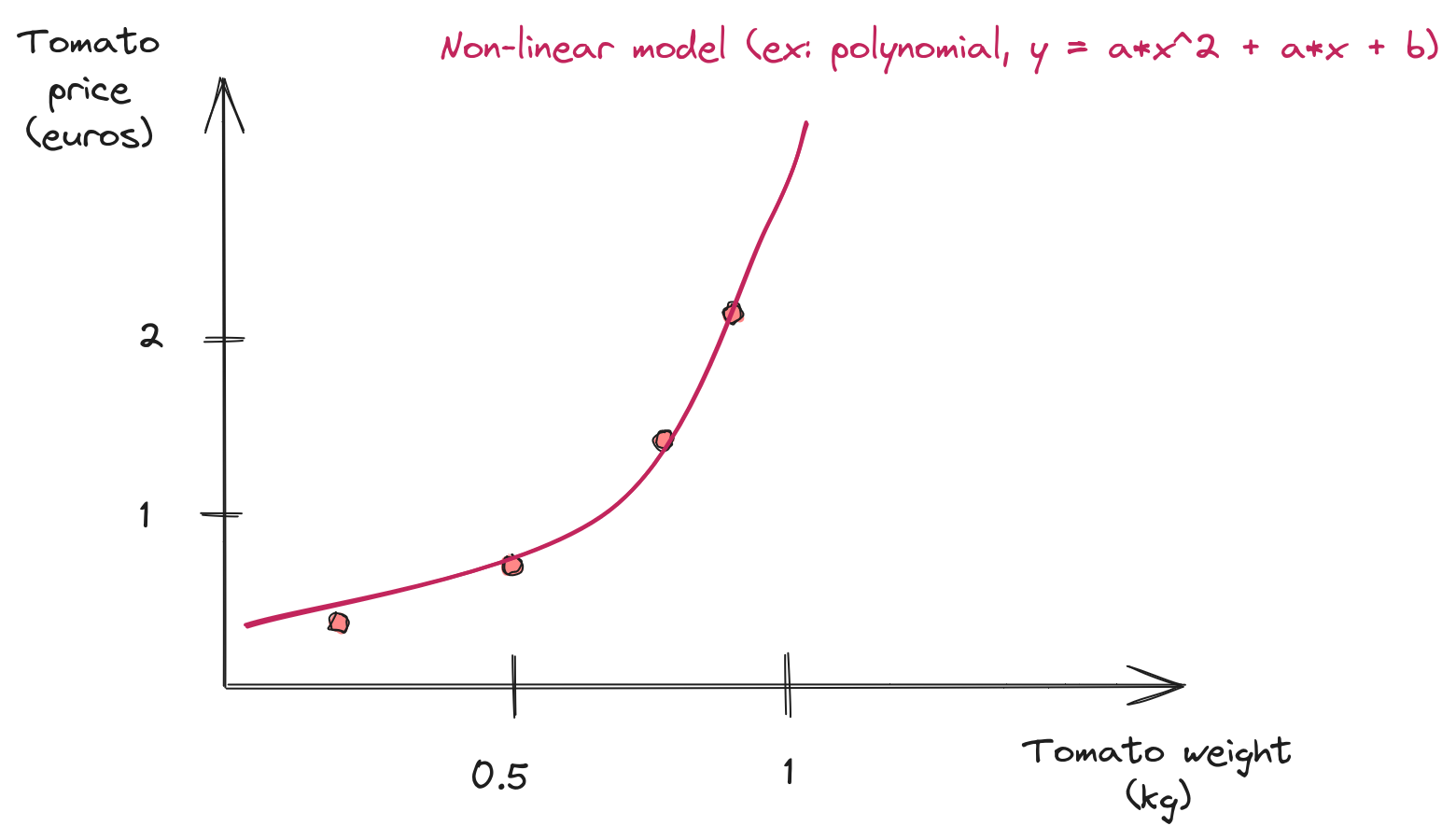### Scikit-Learn API


import numpy as np
from sklearn.linear_model import LinearRegression
# Generate some data (noisy linear function)
x = np.linspace(0, 5, num=50).reshape(50, 1)
y = 2 * x + 10 + 0.1 * np.random.rand()
# Fit a linear model using least squares
model = LinearRegression().fit(x, y)
y_predict = model.predict(x)
# Retrieve the optimized parameters
slope, bias = model.coef_, model.intercept_